# Infinity

(diff) ← Older revision | Latest revision (diff) | Newer revision → (diff)

A concept which arose in various branches of mathematics mainly as the antonym to the concept of finiteness. The concept of infinity is used in analytic and geometric theories to denote "improper" or "infinitely-distant" elements, in set theory and in mathematical logic — in the study of "infinite sets" , and in other branches of mathematics.

1) The concept of infinitely-small and infinitely-large variable magnitudes is a fundamental concept in mathematical analysis. The idea which was held prior to the modern approach to the concept of the infinitely small was, to wit, that finite magnitudes were composed of an infinitely-large number of infinitely-small "indivisibles" (cf. Indivisibles, method of), which were not regarded as variables, but rather as constants smaller than any finite magnitude. This idea is an example of an illegitimate separation of the infinite from the finite: The only meaningful procedure is to subdivide finite magnitudes into a without limit increasing number of components which decrease without limit.

2) Infinity also appears in mathematics in an altogether different context, viz., in the form of "improper" , infinitely-distant geometric images (cf. Infinitely-distant elements). For instance, an infinitely-distant point on a straight lineis regarded as a special constant object which is "attached" to the ordinary finite points. However, the inseparable connection between the infinite and the finite is apparent here as well, if only under a projection from a centre situated outside the straight line, in which the straight line passing through the projection centre and parallel to the original straight lineproves to correspond to the infinitely-distant point.

Of a similar character is the completion of the real number system by two "improper" numbers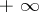and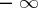, which satisfies many needs in analysis and in the theory of functions of a real variable. The same applies to the completion of the natural number sequence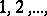by the transfinite numbers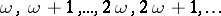(cf. Transfinite number). Owing to the difference between infinitely-small and infinitely-large variables on the one hand, and the "improper" infinitely-large numbers, which are regarded as constants, on the other hand there arose the terms of "potential infinite" (for the former) and "actual infinite" (for the latter). As far as this original concept is concerned (for another, modern, concept see below), the controversy between the protagonists of the potential and the actual infinity may be regarded as settled. Infinitely-small and infinitely-large magnitudes, which are the base of the definition of the derivative (as a ratio of infinitely-small magnitudes) and of the integral (as a sum of an infinitely-large number of infinitely-small magnitudes) are thus related to concepts of mathematical analysis, and should be considered as "potential" . At the same time, the logical framework of mathematics quite legitimately contains "actually infinite" , large "improper" numbers (such numbers are even introduced from several different points of view: as quantitative and ordinal transfinite numbers in set theory, as the improper elementsandinto the real number system, etc.).

Two ways of adding infinite "improper" elements to the number system are usually encountered in mathematics.

a) From the projective point of view, one single "infinitely-distant point" is found on a straight line. In an ordinary metric coordinate system it is natural to assign to this point the abscissa. Such an addition of one infinity, without sign, to the number system is needed in the theory of functions of a complex variable (cf. also Riemann sphere). In elementary analysis, when studying rational functions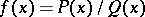, whereand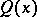are polynomials, it is natural to put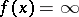at the points at whichhas a zero of higher order than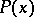.

The following rules for the mathematical operations are defined for the improper element: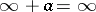ifis finite;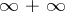has no meaning;if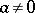;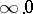has no meaning.

Inequalities involvingare not considered; the question as to whetheris larger or smaller than a finite numberis meaningless.

In the study of real functions of a real variable, the real number system is completed by two improper elements:and. One may then assume that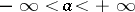for any finite number, and preserve the fundamental properties of inequalities in the enlarged number system. The following rules of arithmetical operations are laid down forand:if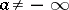;if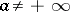;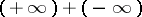is meaningless;if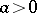;if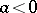;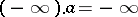if;if;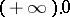and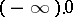are meaningless.

3) The main interest, but also the major difficulty in the mathematical study of infinity, is in the question of the nature of infinite sets of mathematical objects. In particular, it should be borne in mind that the entire abstraction and completeness of the theory of infinitely-small and infinitely-large variables which has been attained so far only means that all difficulties of this theory have been reduced to a question in the foundations of number theory, in which the concept of an infinite system of numbers forms an intrinsic part. To say thatis infinitely small is only meaningful if the nature of the variation ofas a function of some other variableis described; for instance, one says thatis infinitely small as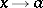if for anythere exists a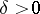such thatif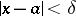. This definition itself is based on the assumption that the function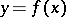is defined for an infinite set of values of(e.g. for all realwhich are sufficiently close to).

In the theory of sets the terms "actual" and "potential" infinite usually have a profound meaning, which has nothing in common with naming an infinite cardinal an "actually infinite number" . This is because infinite systems of mathematical objects (such as the natural or real number system) can never be defined by simple enumeration, as in the case of finite systems of objects. It would obviously be absurd for anyone to claim to have "formed" the set of natural numbers by actually counting "all" of them in succession. In fact, the study of the set of natural numbers is based on the formation of its elements by transition fromto. In the case of the continuum of real numbers, even the study of one of its elements — a real number — leads to the study of the formation of its successive approximate values, while the study of the entire set of real numbers involves the study of the general properties of such formation processes for its elements. In this sense, the infinity of the natural number system or of the real number system (the continuum) may be denoted as a merely "potential" infinity. A point of view which is opposite to potential infinity considers infinite sets as "actually" defined, whatever the process of their formation. The question of justification of such a separation between infinite sets themselves and the process of their formation, and of the conditions under which such an abstraction is justified in their study, is still open. See also Abstraction of actual infinity; Abstraction of potential realizability.